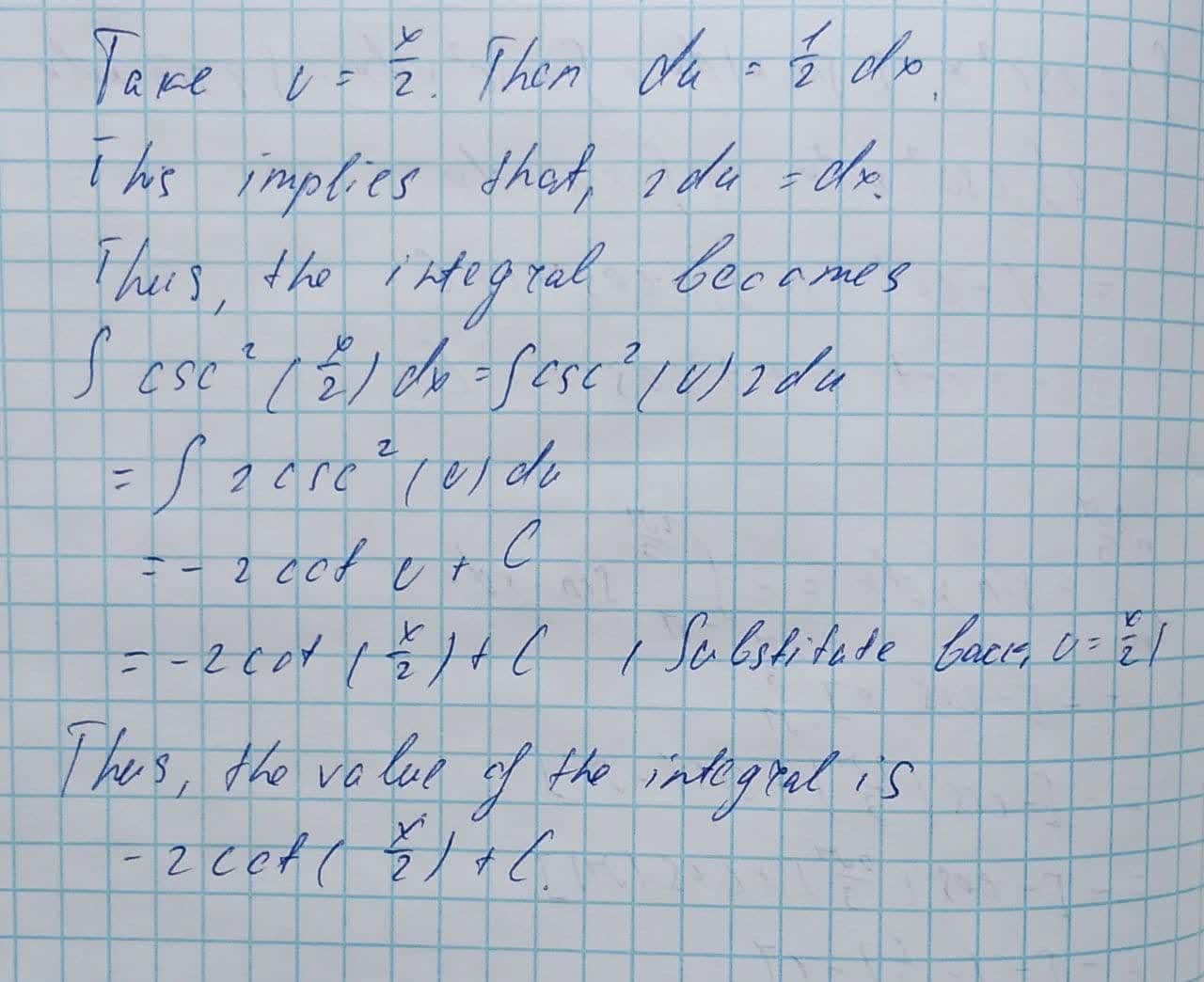Find the indefinite integral: \int \csc^{2}(\frac{x}{2})dxabondantQ 2021-08-06 Answered
Find the indefinite integral.
$$\displaystyle\int{{\csc}^{{{2}}}{\left({\frac{{{x}}}{{{2}}}}\right)}}{\left.{d}{x}\right.}$$

• Questions are typically answered in as fast as 30 minutes

Solve your problem for the price of one coffee

• Math expert for every subject
• Pay only if we can solve itdoplovif
To find the indefinite integral.